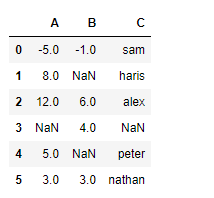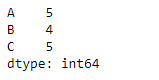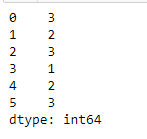# Python | Pandas dataframe.count()

• Difficulty Level : Easy
• Last Updated : 20 Nov, 2018

Python is a great language for doing data analysis, primarily because of the fantastic ecosystem of data-centric python packages. Pandas is one of those packages and makes importing and analyzing data much easier.

Pandas` dataframe.count()` is used to count the no. of non-NA/null observations across the given axis. It works with non-floating type data as well.

Attention geek! Strengthen your foundations with the Python Programming Foundation Course and learn the basics.

To begin with, your interview preparations Enhance your Data Structures concepts with the Python DS Course. And to begin with your Machine Learning Journey, join the Machine Learning - Basic Level Course

Syntax: DataFrame.count(axis=0, level=None, numeric_only=False)

Parameters:
axis : 0 or ‘index’ for row-wise, 1 or ‘columns’ for column-wise
level : If the axis is a MultiIndex (hierarchical), count along a particular level, collapsing into a DataFrame
numeric_only : Include only float, int, boolean data

Returns: count : Series (or DataFrame if level specified)

Example #1: Use `count()` function to find the number of non-NA/null value across the row axis.

 `# importing pandas as pd``import` `pandas as pd`` ` `# Creating a dataframe using dictionary``df ``=` `pd.DataFrame({``"A"``:[``-``5``, ``8``, ``12``, ``None``, ``5``, ``3``], ``                   ``"B"``:[``-``1``, ``None``, ``6``, ``4``, ``None``, ``3``],``                   ``"C:["``sam``", "``haris``", "``alex``", np.nan, "``peter``", "``nathan"]})`` ` `# Printing the dataframe``df`Now find the count of non-NA value across the row axis

 `# axis = 0 indicates row``df.count(axis ``=` `0``)`

Output :Example #2: Use `count()` function to find the number of non-NA/null value across the column.

 `# importing pandas as pd``import` `pandas as pd`` ` `# Creating a dataframe using dictionary``df ``=` `pd.DataFrame({``"A"``:[``-``5``, ``8``, ``12``, ``None``, ``5``, ``3``],``                   ``"B"``:[``-``1``, ``None``, ``6``, ``4``, ``None``, ``3``], ``                   ``"C:["``sam``", "``haris``", "``alex``", np.nan, "``peter``", "``nathan"]})`` ` `# Find count of non-NA across the columns``df.count(axis ``=` `1``)`

Output :My Personal Notes arrow_drop_up